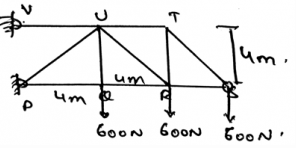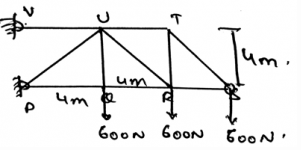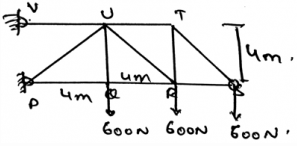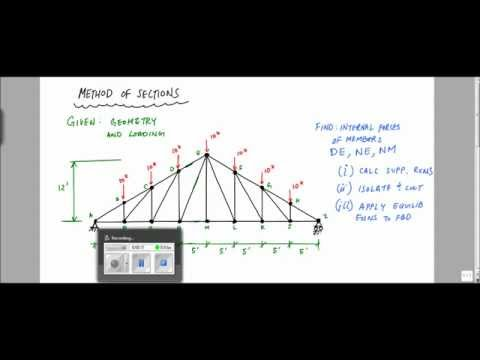Courses

# Test: Method Of Sections

## 15 Questions MCQ Test Engineering Mechanics | Test: Method Of Sections

Description
This mock test of Test: Method Of Sections for Mechanical Engineering helps you for every Mechanical Engineering entrance exam. This contains 15 Multiple Choice Questions for Mechanical Engineering Test: Method Of Sections (mcq) to study with solutions a complete question bank. The solved questions answers in this Test: Method Of Sections quiz give you a good mix of easy questions and tough questions. Mechanical Engineering students definitely take this Test: Method Of Sections exercise for a better result in the exam. You can find other Test: Method Of Sections extra questions, long questions & short questions for Mechanical Engineering on EduRev as well by searching above.
QUESTION: 1

### We use the method of joints when the members on which the force to be found are few in number.

Solution:

For few members we use method of joints to find the unknown force. It defines as if the whole truss is in equilibrium then all the joints which are connected to that truss is in equilibrium. This is known as method of joints. But when the system becomes complex, method of section comes into the picture.

QUESTION: 2

### The method of section cuts the whole structure of trusses into section and then uses the cut out portion for the calculations.

Solution:

The method of section cuts the whole structure of trusses into section and then uses the cut out portion for the calculations. In which the net force equals zero equation is used. The net moment is made to be zero, etc. All these conditions of equilibrium is applied.

QUESTION: 3

### The method of joints helps us to find out the unknown force in the trusses joint. And the method of section helps us to make the calculation of the direction of the zero members.

Solution:

Method of joints defines as if the whole truss is in equilibrium then all the joints which are connected to that truss is in equilibrium. While the method of section cuts the whole structure of trusses into section and then uses the cut out portion for the calculations of the unknown forces. Thus both the methods are used for the same purpose.

QUESTION: 4

Method of section cuts the whole structure of trusses into section and then uses the cut out portion for the calculations of the unknown forces. For this method, at most two forces must be collinear.

Solution:

Method of section cuts the whole structure of trusses into section and then uses the cut out portion for the calculations of the unknown forces. For this method, we don’t require two forces to be collinear. But if the forces are collinear than the task of determining the unknown forces is very easy.

QUESTION: 5

The determination of the truss’s support reaction is done first and then the section is isolated for the method of the section.

Solution:

The determination of the force of the unknown element is done by the method of section. For this the section is isolated from the main structure and then calculation is done after. But before that the determination of the support reaction needs to be done.

QUESTION: 6

Why the determination of the support reaction needs to be done before isolating the section?

Solution:

The determination of the force of the unknown element is done by the method of section. For this the section is isolated from the main structure and then calculation is done after. But before that the determination of the support reaction needs to be done.

QUESTION: 7

The free diagram of the section of the truss is made for the section having the least number of the forces. This is done as to make the calculations easy.

Solution:

The part of the calculation is made easier if the flow diagram of that section is made which is having the least number of forces. Thus for the sake of the calculation this is done. Or one can say that for getting the unknown force we use this method.

QUESTION: 8

We have three method to solve for the unknown force in the trusses. They are method of section, method of joints and rotational vector method.

Solution:

The main methods for the solving for the unknown force on the truss are method of section and the method of joints. The other method is just given for the sake of confusion. This means that only two main methods are to applied for solving the unknown force on truss.

QUESTION: 9

The free body diagram of which part of the section of the truss is made to make use of method of sections?

Solution:

The free body diagram of any part of the section of the truss is made to make use of method of sections. Various equations of equilibrium are made to do the calculations. The net force acting on the vertical direction is made zero. Also horizontal direction forces are made to be zero.

QUESTION: 10

Determine the force in members UT.Solution:

The summation of the forces needs to be zero. So does the summation of the moments need to zero. But talking about the angles, they not needed to zero. But the forces which are acting at particular angles, must needed to be equal to zero. The basic need of the forces to make the body in equilibrium.

QUESTION: 11

Determine the force in members UR.Solution:

The summation of the forces needs to be zero. So does the summation of the moments need to zero. But talking about the angles, they not needed to zero. But the forces which are acting at particular angles, must needed to be equal to zero. The basic need of the forces to make the body in equilibrium.

QUESTION: 12

Determine the force in members QR.Solution:

The summation of the forces needs to be zero. So does the summation of the moments need to zero. But talking about the angles, they not needed to zero. But the forces which are acting at particular angles, must needed to be equal to zero. The basic need of the forces to make the body in equilibrium.

QUESTION: 13

The system of the collinear and the parallel force are simplified as:

Solution:

The simplification is easy. The simplified force system gives us a net force and the parallel force system gives us a simplified force, and then we add it vectorially. Thus the vector addition at the last can be easily done and thus both can be simplified.

QUESTION: 14

The couple and the other two force systems can be easily simplified.

Solution:

Both of them are vector quantities. And both of them can be easily simplified. If taken in the vector form then the task is even easier. Thus it is not necessary for the force or the couple to be vector only, even if the magnitude is taken, the simplification is done in the 2D.

QUESTION: 15

The resultant force is equal to the _______ of all the forces.

Solution:

As the simplification means addition and the subtraction of the forces. This results in the simplification of the forces and thus gives us a single force. It is in a particular direction. But if one is considering the direction with the magnitude of the force, we can say that the resultant is the sum of all the forces.

Track your progress, build streaks, highlight & save important lessons and more!

### Similar Content### Related tests